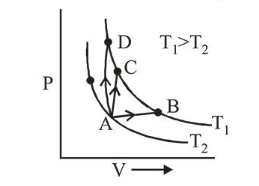# Three different processes that can occur in an ideal monoatomic gas`
Question:

Three different processes that can occur in an ideal monoatomic gas are shown in the $\mathrm{P}$ vs $\mathrm{V}$ diagram. The paths are lebelled as $\mathrm{A} \rightarrow \mathrm{B}, \mathrm{A} \rightarrow \mathrm{C}$ and $\mathrm{A} \rightarrow \mathrm{D}$. The change in internal energies during these process are taken as $E_{A B}$, $E_{\mathrm{AC}}$ and $E_{\mathrm{AD}}$ and the workdone as $W_{\mathrm{AB}}, W_{\mathrm{AC}}$ and $W_{\mathrm{AD}}$. The correct relation between these parameters are :1. $\mathrm{E}_{\mathrm{AB}}=\mathrm{E}_{\mathrm{AC}}<\mathrm{E}_{\mathrm{AD}}, \mathrm{W}_{\mathrm{AB}}>0, \mathrm{~W}_{\mathrm{AC}}=0, \mathrm{~W}_{\mathrm{AD}}<0$

2. $\mathrm{E}_{\mathrm{AB}}=\mathrm{E}_{\mathrm{AC}}=\mathrm{E}_{\mathrm{AD}}, \mathrm{W}_{\mathrm{AB}}>0, \mathrm{~W}_{\mathrm{AC}}=0, \mathrm{~W}_{\mathrm{AD}}>0$

3. $\mathrm{E}_{\mathrm{AB}}<\mathrm{E}_{\mathrm{AC}}<\mathrm{E}_{\mathrm{AD}}, \mathrm{W}_{\mathrm{AB}}>0, \mathrm{~W}_{\mathrm{AC}}>\mathrm{W}_{\mathrm{AD}}$

4. $\mathrm{E}_{\mathrm{AB}}>\mathrm{E}_{\mathrm{AC}}>\mathrm{E}_{\mathrm{AD}}, \mathrm{W}_{\mathrm{AB}}<\mathrm{W}_{\mathrm{AC}}<\mathrm{W}_{\mathrm{AD}}$

Correct Option: , 2

Solution:

(2) Temperature change $\Delta T$ is same for all three

processes $A \rightarrow B ; A \rightarrow C$ and $A \rightarrow D$

$\Delta U=n C_{v} \Delta T=$ same

$E_{A B}=E_{A C}=E_{A D}$

Work done, $W=P \times \Delta V$

$A B \rightarrow$ volume is increasing $\Rightarrow W_{A B}>0$

$A D \rightarrow$ volume is decreasing $\Rightarrow W_{A D}<0$

$A C \rightarrow$ volume is constant $\Rightarrow W_{A C}=0$